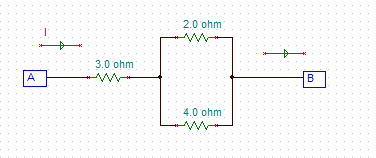# hree resistors are connected as shown in the figure. The potential difference between points A...

## Question:

Three resistors are connected as shown in the figure. The potential difference between points {eq}A \ and \ B {/eq} is {eq}26 \ V. {/eq} How much current flows through the {eq}3 \ \Omega {/eq} resistor?## Ohm's Law:

When we know any two, the voltage V, current I, or resistance R of a branch in an electric circuit, we can get the third parameter using Ohm's Law which relates the three. According to the law, {eq}I = \frac{V}{R} {/eq}.

The equivalent resistance R of the branch shown is {eq}R = 3 + 2||4 = 3 +\frac{2(4)}{2+4} = 4.33 \ \Omega {/eq}

The potential difference equals...

Become a Study.com member to unlock this answer! Create your account